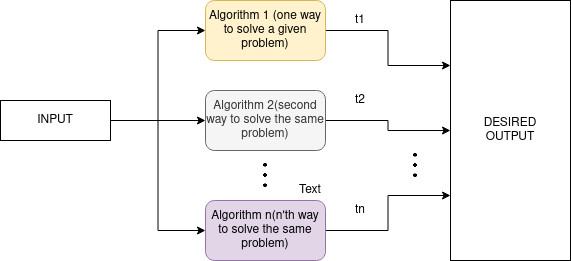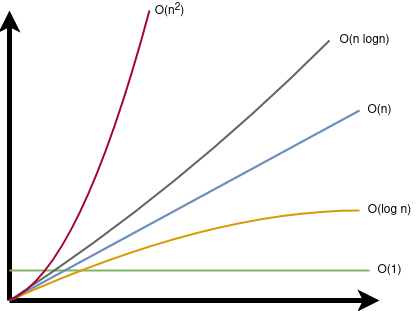# Time and Space Complexity In Algorithms, Beginners GuideMultiple algorithms with different implementation to solve single problem.

# Asymptotic Notations

Asymptotic notation is a way to calculate the algorithm’s behavior for time complexity in its three input types that are best, worst, and average. What do I mean by this, Well the algorithm behaves Differently when the given input is engineered. forex. In bubble sort, sorted data will give the best time complexity than its counterpart where the data is sorted but is in reverse order.Big Oh(O) with different time complexity.

# Space and Time Complexity

Time complexity is a measure in the unit of how much time it will take for an algorithm to solve a certain problem given an n number of inputs. Here time is not the normal clock time that we are used to, but it’s a unit for the execution of 1 instruction. that is how many instructions the CPU must execute to solve a problem. Well, the lessor time is taken by the algorithm is better because it is efficient and cheap in terms of CPU cycles. If an algorithm takes around n unit time to process n inputs in the worst case. Then the time complexity for that algorithm is O(n). Diagram in the above section shows different time complexities which algorithm can have.

# Example of Time and Space Complexity

`public int linearSearch(String[] arr, String element) {  for (int i = 0; i < arr.length; i++) {   if(arr[i].equals(element)) {    return i;   }   }  return -1; }`

# Conclusion

In the end space and time complexity is a topic of research in itself and can be learned for years. hence the aim of this blog is only to give its reader basic understanding of this topic.

--

--